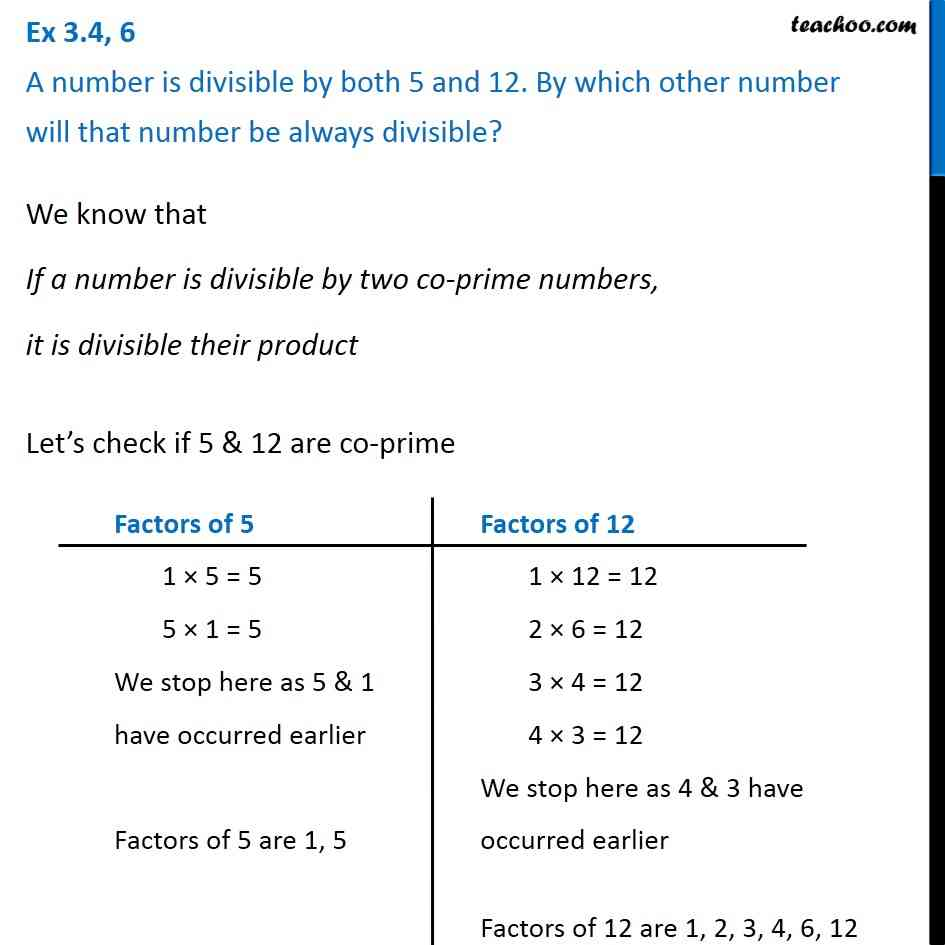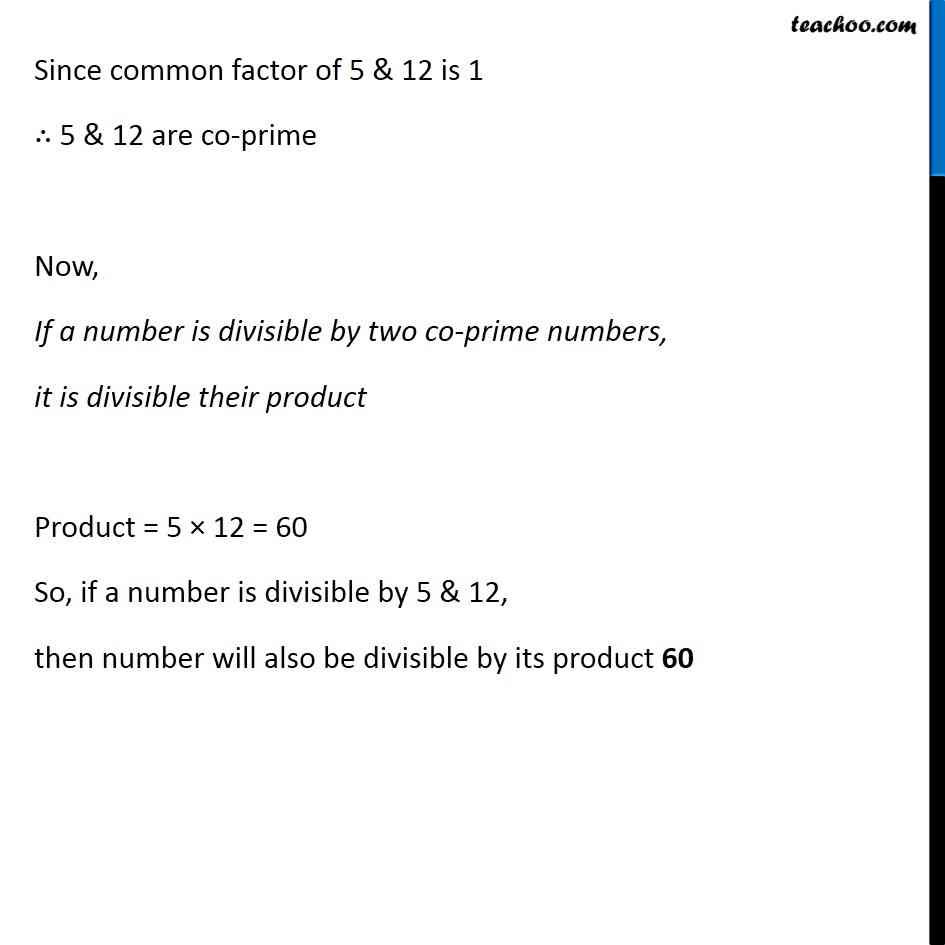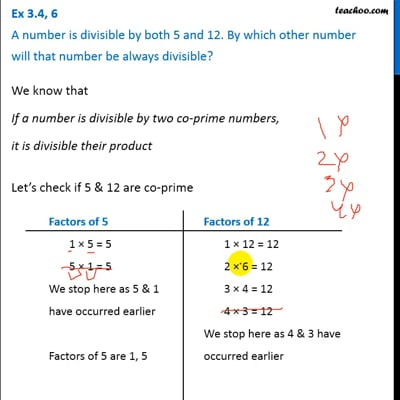More Divisibility Rules

Chapter 3 Class 6 Playing with Numbers
Concept wiseThis video is only available for Teachoo black users

### Transcript

Ex 3.4, 6 A number is divisible by both 5 and 12. By which other number will that number be always divisible? We know that If a number is divisible by two co-prime numbers, it is divisible their product Let’s check if 5 & 12 are co-prime Factors of 5 1 × 5 = 5 5 × 1 = 5 We stop here as 5 & 1 have occurred earlier Factors of 5 are 1, 5 Factors of 12 1 × 12 = 12 2 × 6 = 12 3 × 4 = 12 4 × 3 = 12 We stop here as 4 & 3 have occurred earlier Factors of 12 are 1, 2, 3, 4, 6, 12 Since common factor of 5 & 12 is 1 ∴ 5 & 12 are co-prime Now, If a number is divisible by two co-prime numbers, it is divisible their product Product = 5 × 12 = 60 So, if a number is divisible by 5 & 12, then number will also be divisible by its product 60